# Liouville-equation(2)

(diff) ← Older revision | Latest revision (diff) | Newer revision → (diff)

The equation of motion for the distribution function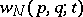with respect to the momenta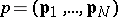and coordinates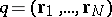of the-particle classical system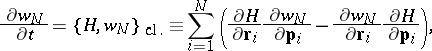whereis the Hamiltonian of the system, and the curly brackets denote the classical Poisson brackets.

In the phase space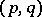the distributionis associated with the density of the phase points (each of which corresponds to a definite mechanical state of the given system ofmaterial points). By virtue of the fact that the trajectories of the motion of these points do not intersect, because of the uniqueness of the solutions of the mechanical equations of motion, and the fact that the phase volume is conserved, by the Liouville theorem (cf. Liouville theorems 4)), the ensemble of these points forms in the phase space a kind of incompressible liquid. The complete derivative of its density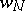with respect to time is equal to zero: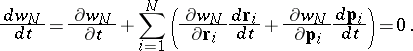This leads to Liouville's equation if, in accordance with the Hamilton equations, one expresses the derivatives of the coordinates and momenta in terms of the corresponding partial derivatives of the Hamiltonian.

Liouville's equation is used not only in the examination of general questions of statistical mechanics connected with the determination of the microscopic and macroscopic structures of the state of a system of several bodies, processes of tending towards equilibrium, problems of "mixing" in phase space, ergodicity, etc., but also in specific investigations, since Liouville's equation is the original equation for the construction of the Bogolyubov chain of equations, and consequently also for a different type of kinetic equation, by means of which problems of applied physics are solved.

In the case of quantum systems the role of Liouville's equation is played by the equation of motion for the statistical operator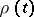(the density matrix), which in the Schrödinger representation has the form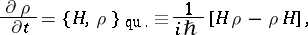whereis the Hamilton operator and the curly brackets denote the Poisson quantum bracket. This quantum Liouville equation is a consequence of the structure of the mixed state (described by a given statistical operator), in which each of the pure quantum-mechanical states comprising it evolves according to the Schrödinger equation.

How to Cite This Entry:
Liouville-equation(2). Encyclopedia of Mathematics. URL: http://encyclopediaofmath.org/index.php?title=Liouville-equation(2)&oldid=13598
This article was adapted from an original article by I.A. Kvasnikov (originator), which appeared in Encyclopedia of Mathematics - ISBN 1402006098. See original article